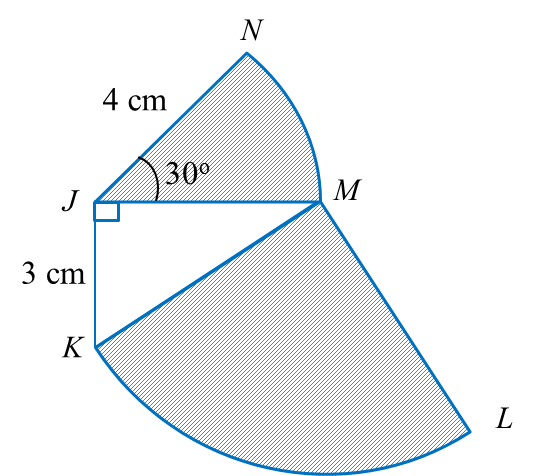# 10.2.5 Perimeter and Area, PT3 Practice

 
 
Question 12:
Diagram shows a quadrant KLM with centre  M and sector JMN with centre J.Using           π    =             22         7             , calculate
    
(a) the perimeter, in cm, of the whole diagram,
 
(b) the area, in cm2, of the shaded region.
 
 
[6 marks]
 
 
Solution:
 
(a)
 
KM2 = JK2 + JM2
 
KM2 = 32+ 42
 
KM2 = 25
 
KM = 5 cm
 
 
Perimeter of the whole diagram
 
= NJ + JK + KL+ LM + MN
                   =     4     +     3     +         (                1      4           ×      2      ×                  22            7           ×      5          )         +     5     +         (                       30                   360                 ×      2      ×                  22            7           ×      4          )                       =     7     +         (                       55            7               )         +     5     +         (                       44                   21                     )                       =     21               20                21                  c     m              
 
 

  (b)
 
 
  
                   =           (                  1       4            ×                   22             7            ×             5       2                 )          +           (                        30                   360                  ×                   22             7            ×             4       2                 )                          =                274                14               +                88                21                               =     23                16                21                    c           m      2